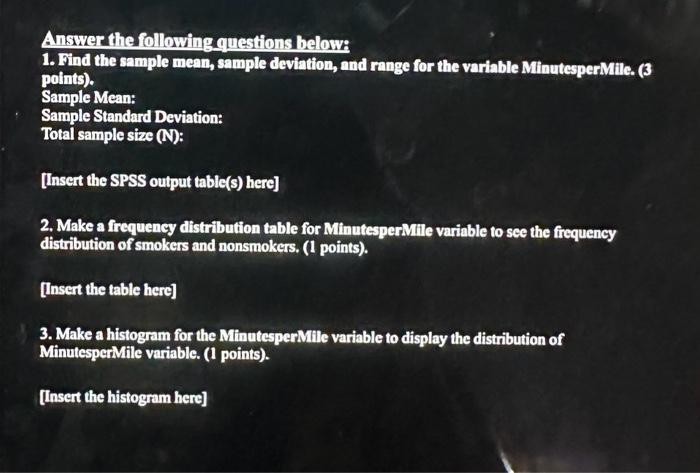# (Solved): Answer the following questions belowi 1. Find the sample mean, sample deviation, and range for the ...Answer the following questions belowi 1. Find the sample mean, sample deviation, and range for the variable MinutesperMile. (3 points). Sample Mean: Sample Standard Deviation: Total sample size (N): [Insert the SPSS output table(s) here] 2. Make a frequency distribution table for MinutesperMille variable to see the frequency distribution of smokers and nonsmokers. (1 points). [Insert the table here] 3. Make a histogram for the MinutesperMile variable to display the distribution of MinutesperMile variable. (1 points). [Insert the histogram here]

We have an Answer from Expert